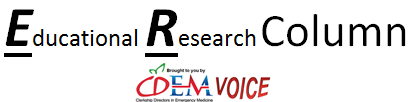Educational Research Column: Choosing Wisely: Chi-Square vs. Fisher’s Exact

Dec 17, 2018, 13:52 PM by Nick Olah
Choosing the ideal statistical test will help get to the true answer. Much like in our clinical practice, where we have to weigh the risks and benefits of diagnostic testing, the same holds true in statistical testing. Every test has its limitations and risk of giving a false positive or negative.Choosing Wisely: Chi-Square vs. Fisher's Exact

Choosing the ideal statistical test will help get to the true answer. Much like in our clinical practice, where we have to weigh the risks and benefits of diagnostic testing, the same holds true in statistical testing. Every test has its limitations and risk of giving a false positive or negative. That is why it is important to choose the optimum test.

In educational research, we often find ourselves analyzing data arranged in a contingency table, and then have to choose the “right” test.  Both the Fisher’s exact and Chi-square test can be used.  In order to choose the best test for your data we must understand how the tests work and their limitations.

The chi-square test for independence compares variables in a contingency table.  It is a particularly useful statistic because in addition to determining whether a significant difference is observed it also helps to identify which categories are responsible for those differences.  As a non-parametric test, it does not require assumptions about the distribution the data is drawn from, but does have its own requirements that must be met for a useful and valid result.

To use a chi-square test, the data should be count or frequencies rather than percentages of sufficiently large sample size.  The categories used must be mutually exclusive (for example intervention vs. control group), must be independent and not a paired sample.   There can be only two variables, however for each variable there can be multiple levels (for example the 5-level Likert scale).  Finally, there must be an expected minimum count of at least 5 in at least 80% of the cells in the table.  For instance, in a 2×2 contingency table if one of the four categories has an expected count of less than 5, the chi-square test becomes unreliable. A good rule of thumb is that if the sample size is at least five times the number of cells this should satisfy the final assumption.

While the chi-square is a very useful test to determine if a significant difference is observed, it does not provide much information about the strength or magnitude of the difference.  If a sample size is large enough we can achieve statistical significance even though there is little strength to the association.  To determine the strength of the association a test such as Cramer’s V can be applied.  In addition to the fact that a sufficiently large sample size can yield statistical significance, the chi-square test is also sensitive to small frequencies.  If the expected frequencies in cells are below 5, or more than 20% of cells are below five, the method of approximation used to calculate the chi-square becomes unreliable and risks either a type I or type II error.

The scenario of low expected cell frequencies may be encountered in small sample size educational research or clinical trials.  This is where the Fisher’s Exact test is superior.  The Fisher’s exact test is just that, exact.  It does not use an approximation like the chi-square test and therefore remains valid for small sample sizes.  When the sample size becomes large enough the p-value generated from a chi-square will approach that of a Fisher’s exact.  Fisher’s exact also has the benefit of being valid at large sample sizes.

Historically, statistical tests using approximations such as the chi-square were used because of the arduous calculations required for exact tests.  Now with powerful computers these calculations are easy to perform and generate exact values and do share as significant a risk of type I or type II error due to small sample size.  While typically only used for 2×2 tables, Fisher’s exact can be used with larger contingency tables provided you have ample computing power.

Jason J. Lewis, MD & David Schoenfeld, MD, MPH
Beth Israel Deaconess Medical Center/Harvard Medical School